SSC (English Medium) Class 8Maharashtra State Board
Share

# Balbharati solutions for Class 8 Mathematics chapter 15 - Area

#### Balbharati Balbharati Class 8 Mathematics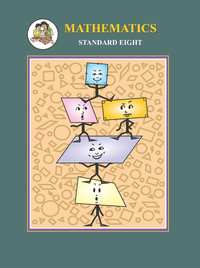## Chapter 15: Area

Practice Set 15.1Practice Set 15.2Practice Set 15.3Practice Set 15.4Practice Set 15.5Practice Set 15.6

#### Chapter 15: Area Exercise Practice Set 15.1 solutions [Page 95]

Practice Set 15.1 | Q 1 | Page 95

If base of a parallelogram is 18 cm and its height is 11 cm, find its area.

Practice Set 15.1 | Q 2 | Page 95

If area of a parallelogram is 29.6 sq cm and its base is 8 cm, find its height.

Practice Set 15.1 | Q 3 | Page 95

Area of a parallelogram is 83.2 sq cm. If its height is 6.4 cm, find the length of its base.

#### Chapter 15: Area Exercise Practice Set 15.2 solutions [Page 97]

Practice Set 15.2 | Q 1 | Page 97

Lengths of the diagonals of a rhombus are 15 cm and 24 cm, find its area.

Practice Set 15.2 | Q 2 | Page 97

Lengths of the diagonals of a rhombus are 16.5 cm and 14.2 cm, find its area.

Practice Set 15.2 | Q 3 | Page 97

If perimeter of a rhombus is 100 cm and length of one diagonal is 48 cm, what is the area of the quadrilateral ?

Practice Set 15.2 | Q 4 | Page 97

If length of a diagonal of a rhombus is 30 cm and its area is 240 sq cm, find its perimeter.

#### Chapter 15: Area Exercise Practice Set 15.3 solutions [Page 99]

Practice Set 15.3 | Q 1 | Page 99

In ☐ ABCD, l(AB) = 13 cm, l(DC) = 9 cm, l(AD) = 8 cm, find the area of ☐ ABCD.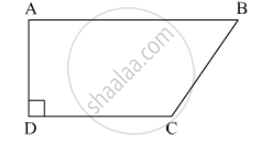Practice Set 15.3 | Q 2 | Page 99

Length of the two parallel sides of a trapezium are 8.5 cm and 11.5 cm respectively and its height is 4.2 cm, find its area.

Practice Set 15.3 | Q 3 | Page 99

☐ PQRS is an isosceles trapezium l(PQ) = 7 cm. seg PM ⊥ seg SR, l(SM) = 3 cm, Distance between two parallel sides is 4 cm, find the area of ☐ PQRS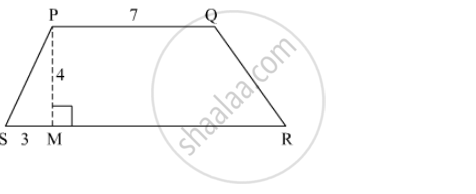#### Chapter 15: Area Exercise Practice Set 15.4 solutions [Page 101]

Practice Set 15.4 | Q 1 | Page 101

Sides of a triangle are cm 45 cm, 39 cm and 42 cm, find its area.

Practice Set 15.4 | Q 2 | Page 101

Look at the measures shown in the adjacent figure and find the area of ☐ PQRS.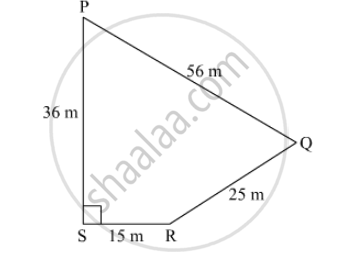Practice Set 15.4 | Q 3 | Page 101

Some measures are given in the adjacent figure, find the area of ☐ABCD.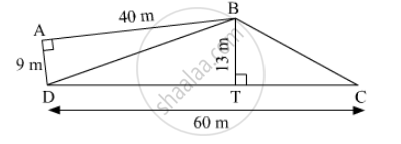#### Chapter 15: Area Exercise Practice Set 15.5 solutions [Page 102]

Practice Set 15.5 | Q 1.1 | Page 102

Find the areas of given plot. (All measures are in metres.)

(1)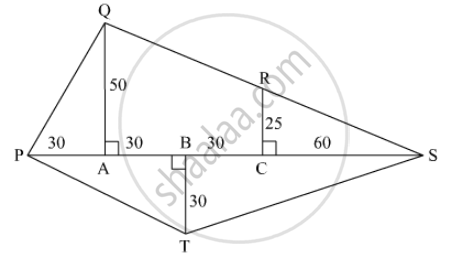Practice Set 15.5 | Q 1.2 | Page 102

Find the areas of given plot. (All measures are in metres.)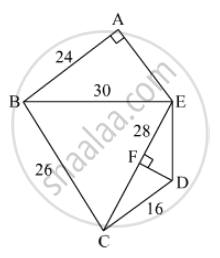#### Chapter 15: Area Exercise Practice Set 15.6 solutions [Pages 0 - 104]

Radii of the circles are given below, find their areas.
(1) 28 cm
(2) 10.5 cm
(3) 17.5 cm

Areas of some circles are given below find their diameters.
(1) 176 sq cm
(2) 394.24 sq cm
(3) 12474 sq cm

Diameter of the circular garden is 42 m. There is a 3.5 m wide road around the garden. Find the area of the road.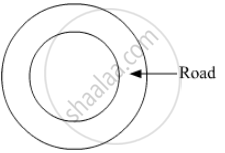Find the area of the circle if its circumfence is 88 cm.

## Chapter 15: Area

Practice Set 15.1Practice Set 15.2Practice Set 15.3Practice Set 15.4Practice Set 15.5Practice Set 15.6

#### Balbharati Balbharati Class 8 Mathematics## Balbharati solutions for Class 8 Mathematics chapter 15 - Area

Balbharati solutions for Class 8 chapter 15 (Area) include all questions with solution and detail explanation. This will clear students doubts about any question and improve application skills while preparing for board exams. The detailed, step-by-step solutions will help you understand the concepts better and clear your confusions, if any. Shaalaa.com has the Maharashtra State Board Balbharati Class 8 Mathematics solutions in a manner that help students grasp basic concepts better and faster.

Further, we at Shaalaa.com provide such solutions so that students can prepare for written exams. Balbharati textbook solutions can be a core help for self-study and acts as a perfect self-help guidance for students.

Concepts covered in Class 8 Mathematics chapter 15 Area are Area of a Parallelogram, Area of a Rhombus, Area of a Trapezium, Introduction to Area of a Triangle, Area of a Triangle by Heron’S Formula, Area of Figures Having Irregular Shape, Perimeter and Area of a Circle.

Using Balbharati Class 8 solutions Area exercise by students are an easy way to prepare for the exams, as they involve solutions arranged chapter-wise also page wise. The questions involved in Balbharati Solutions are important questions that can be asked in the final exam. Maximum students of Maharashtra State Board Class 8 prefer Balbharati Textbook Solutions to score more in exam.

Get the free view of chapter 15 Area Class 8 extra questions for and can use Shaalaa.com to keep it handy for your exam preparation

S